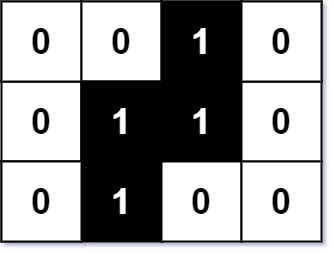### LeetCode• ㊗️
• 大家
• offer
• 多多！

## Problem

You are given an m x n binary matrix image where 0 represents a white pixel and 1 represents a black pixel.

The black pixels are connected (i.e., there is only one black region). Pixels are connected horizontally and vertically.

Given two integers x and y that represents the location of one of the black pixels, return the area of the smallest (axis-aligned) rectangle that encloses all black pixels.

You must write an algorithm with less than O(mn) runtime complexity

Example 1:Input: image = [["0","0","1","0"],["0","1","1","0"],["0","1","0","0"]], x = 0, y = 2
Output: 6


Example 2:

Input: image = [["1"]], x = 0, y = 0
Output: 1


## Code

class Solution {
public int minArea(char[][] image, int x, int y) {
int m = image.length - 1;
int n = image.length - 1;
int leftMost = findLeft(image,0, y, true);
int rightMost = findRight(image, y, n, true);

int topMost = findLeft(image, 0, x, false);
int bottomMost = findRight(image, x, m, false);

return (rightMost - leftMost + 1) * (bottomMost - topMost + 1);
}

private int findLeft(char[][] image, int start, int end, boolean isHor){
int left = start;
int right = end;

while(left + 1 < right){
int mid = left + (right - left) / 2;

if(find(image, mid, isHor)){
right = mid;
} else {
left = mid;
}

}
if(find(image, left, isHor)){
return left;
} else {
return right;
}
}

private int findRight(char[][] image, int start, int end, boolean isHor){
int left = start;
int right = end;

while(left + 1 < right){
int mid = left + (right - left) / 2;

if(find(image, mid, isHor)){
left = mid;
} else{
right = mid;
}
}

if(find(image, end, isHor)){
return right;
} else {
return left;
}
}

private boolean find(char[][] image, int mid, boolean isHor){
if(isHor){
for(int i = 0; i < image.length; i++){
if(image[i][mid] == '1'){
return true;
}
}

return false;
} else {
for(int j = 0; j < image.length; j++){
if(image[mid][j] == '1'){
return true;
}
}

return false;
}
}
}